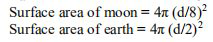# NCERT Solutions Class 9 Mathematics Solutions for Surface Areas and Volumes - Exercise 13.4 in Chapter 13 - Surface Areas and Volumes

The diameter of the moon is approximately one-fourth of the diameter of the earth.

Find the ratio of their surface areas.

If the diameter of the earth is said d, then the diameter of the moon will be d/4 (as per given statement)

Radius of moon = ½ × d/4 = d/8\begin{aligned} &\text { Ratio of their Surface areas }=\frac{4 \pi\left(\frac{d}{8}\right)^{2}}{4 \pi\left(\frac{d}{2}\right)^{2}}=4 / 64=1 / 16\\ &\text { The ratio between their surface areas is } 1: 16 \text { . } \end{aligned}

Video transcript
Related Questions

Lido

Courses

Teachers

Book a Demo with us

Syllabus

Maths
CBSE
Maths
ICSE
Science
CBSE

Science
ICSE
English
CBSE
English
ICSE
Coding

Terms & Policies

Selina Question Bank

Maths
Physics
Biology

Allied Question Bank

Chemistry
Connect with us on social media!